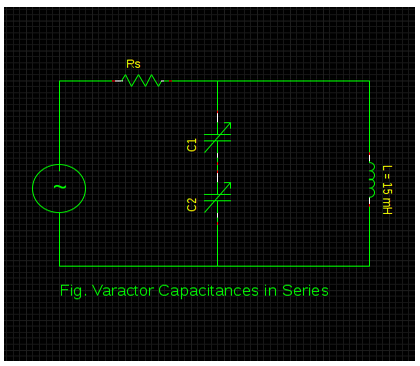# Tuning Range of the circuit that utilizes two varactor diodes with L = 15 mH and capacitance varies

## Determine the tuning range of the following circuit that utilizes two varactor diodes with L = 15 mH and whose capacitance varies from 10 to 70 pF .Options:

a. 318 kHz to 1 MHz
b. 418 kHz to 1 MHz
c. 594 kHz to 1.83MHz
d. 694 kHz to 1.83 MHz

Correct Answer: d. 694 kHz to 1.83 MHz

Explanation:

As per the circuit configuration,

CT ( min) = 10 /2 = 5 pF & CT ( max) = 70 /2 = 35 pF

fr (max) = 1 / 2π (√LC )

= 1 / 2π ( √15 x 10-3 x 5 x 10 -12)

= 1.83 MHz

fr (min) = 1 / 2π (√LC )

= 1 / 2π (√15 x 10-3 x 35 x 10-12)

= 694 kHz